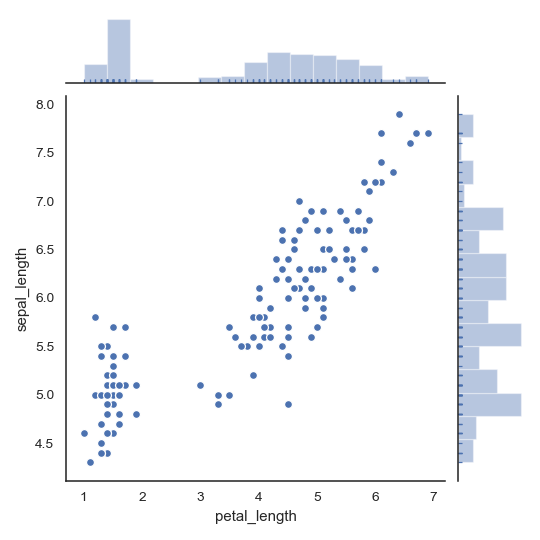# seaborn.jointplot¶

`seaborn.``jointplot`(x, y, data=None, kind='scatter', stat_func=None, color=None, height=6, ratio=5, space=0.2, dropna=True, xlim=None, ylim=None, joint_kws=None, marginal_kws=None, annot_kws=None, **kwargs)

Draw a plot of two variables with bivariate and univariate graphs.

This function provides a convenient interface to the `JointGrid` class, with several canned plot kinds. This is intended to be a fairly lightweight wrapper; if you need more flexibility, you should use `JointGrid` directly.

Parameters
x, ystrings or vectors

Data or names of variables in `data`.

DataFrame when `x` and `y` are variable names.

kind{ “scatter” | “reg” | “resid” | “kde” | “hex” }, optional

Kind of plot to draw.

stat_funccallable or None, optional

Deprecated

colormatplotlib color, optional

Color used for the plot elements.

heightnumeric, optional

Size of the figure (it will be square).

rationumeric, optional

Ratio of joint axes height to marginal axes height.

spacenumeric, optional

Space between the joint and marginal axes

dropnabool, optional

If True, remove observations that are missing from `x` and `y`.

{x, y}limtwo-tuples, optional

Axis limits to set before plotting.

{joint, marginal, annot}_kwsdicts, optional

Additional keyword arguments for the plot components.

kwargskey, value pairings

Additional keyword arguments are passed to the function used to draw the plot on the joint Axes, superseding items in the `joint_kws` dictionary.

Returns
grid`JointGrid`

`JointGrid` object with the plot on it.

`JointGrid`

The Grid class used for drawing this plot. Use it directly if you need more flexibility.

Examples

Draw a scatterplot with marginal histograms:

```>>> import numpy as np, pandas as pd; np.random.seed(0)
>>> import seaborn as sns; sns.set(style="white", color_codes=True)
>>> g = sns.jointplot(x="total_bill", y="tip", data=tips)
```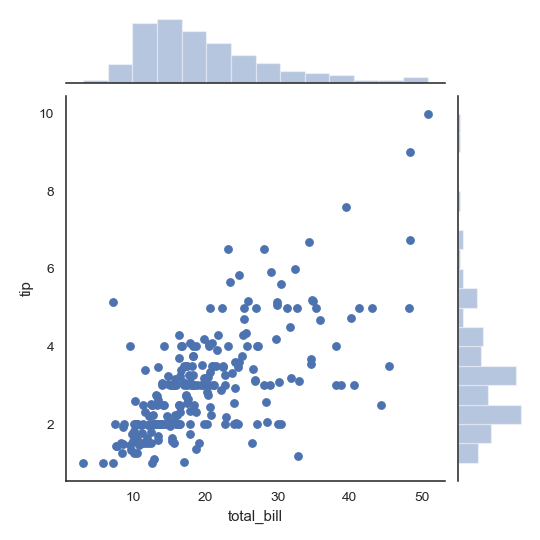Add regression and kernel density fits:

```>>> g = sns.jointplot("total_bill", "tip", data=tips, kind="reg")
```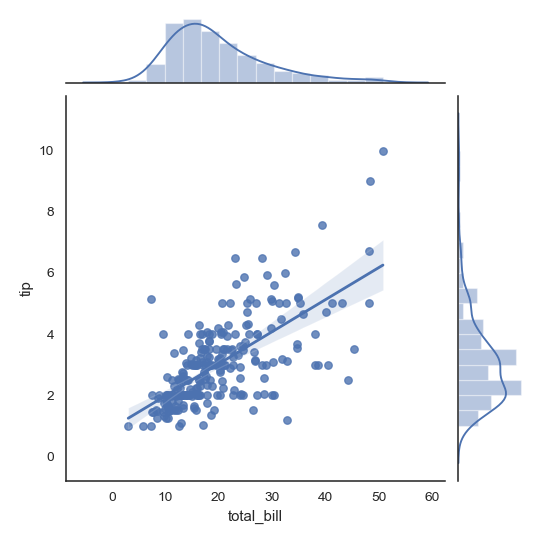Replace the scatterplot with a joint histogram using hexagonal bins:

```>>> g = sns.jointplot("total_bill", "tip", data=tips, kind="hex")
```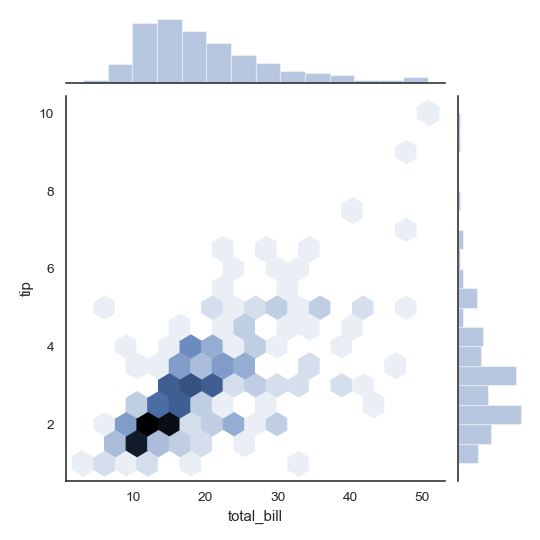Replace the scatterplots and histograms with density estimates and align the marginal Axes tightly with the joint Axes:

```>>> iris = sns.load_dataset("iris")
>>> g = sns.jointplot("sepal_width", "petal_length", data=iris,
...                   kind="kde", space=0, color="g")
```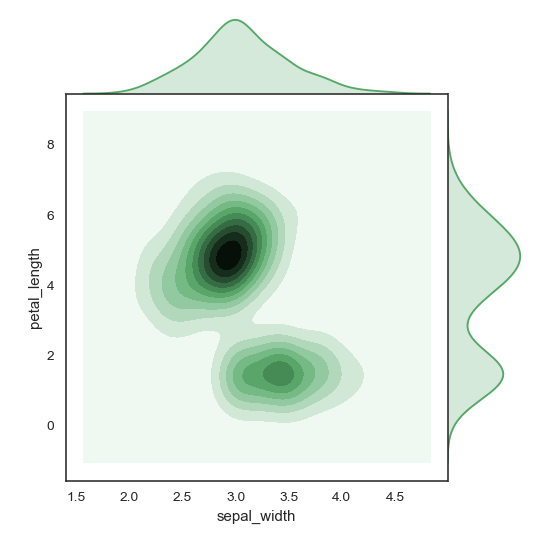Draw a scatterplot, then add a joint density estimate:

```>>> g = (sns.jointplot("sepal_length", "sepal_width",
...                    data=iris, color="k")
...         .plot_joint(sns.kdeplot, zorder=0, n_levels=6))
```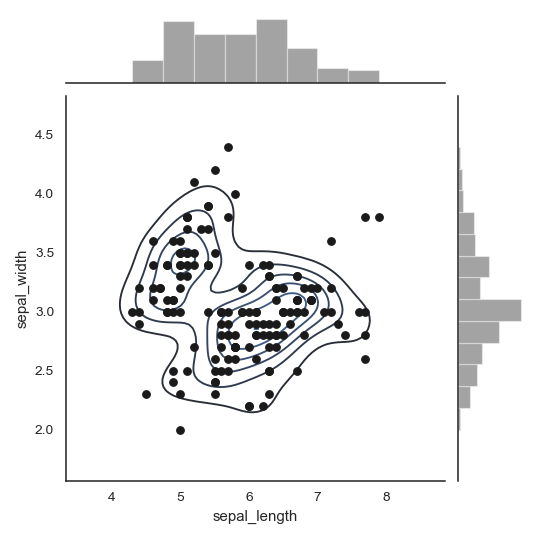Pass vectors in directly without using Pandas, then name the axes:

```>>> x, y = np.random.randn(2, 300)
>>> g = (sns.jointplot(x, y, kind="hex")
...         .set_axis_labels("x", "y"))
```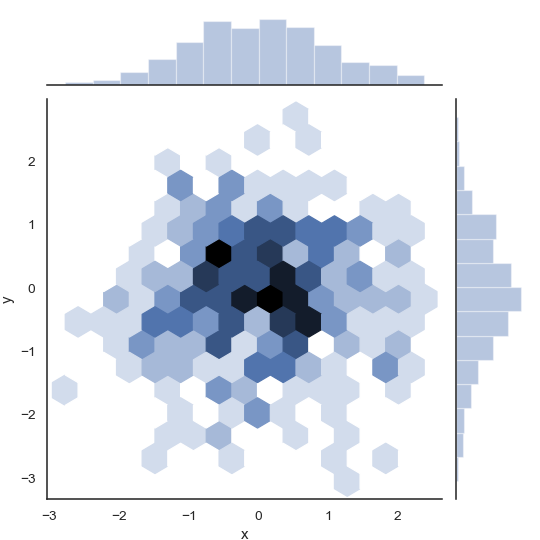Draw a smaller figure with more space devoted to the marginal plots:

```>>> g = sns.jointplot("total_bill", "tip", data=tips,
...                   height=5, ratio=3, color="g")
```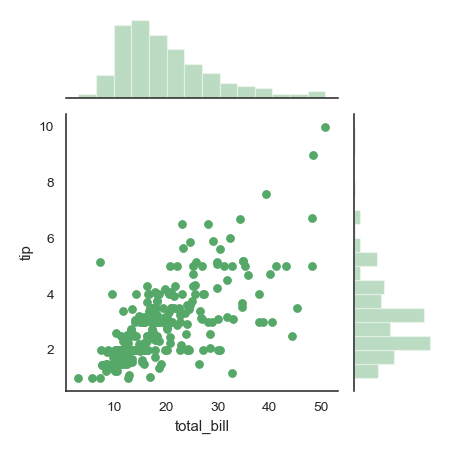Pass keyword arguments down to the underlying plots:

```>>> g = sns.jointplot("petal_length", "sepal_length", data=iris,
...                   marginal_kws=dict(bins=15, rug=True),
...                   annot_kws=dict(stat="r"),
...                   s=40, edgecolor="w", linewidth=1)
```